Latest Banking jobs   »

# Quantitative Aptitude Quiz For SBI Clerk Mains 2022- 13th December

Directions (1-4): Given below line graph shows total number of students take admission for B.TECH course in five different IIT’s in the 2016 & 2017. Read the graph carefully and answer the questions :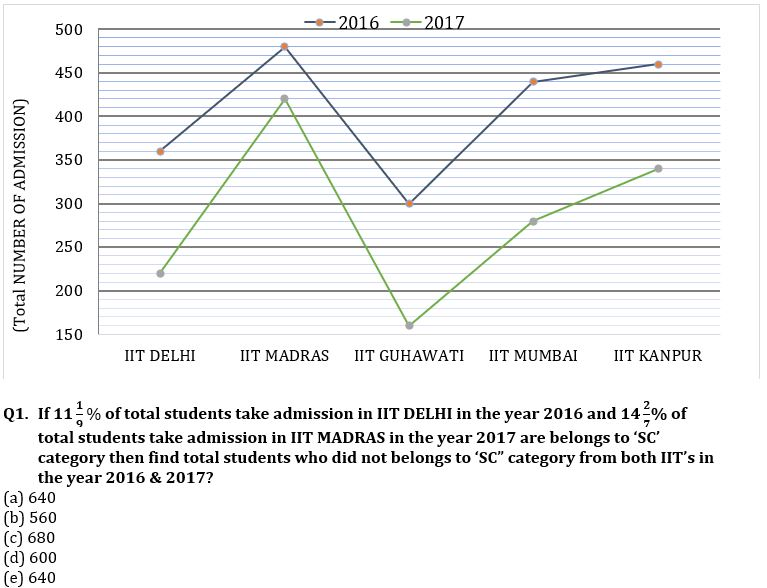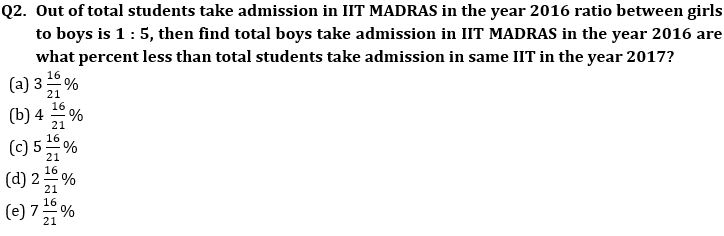Q3. Find the difference between average of number of students take admission in IIT KANPUR in the both years and average of number of students take admission in IIT GUHAWATI in the both years?
(a) 120
(b) 100
(c) 160
(d) 170
(e) 150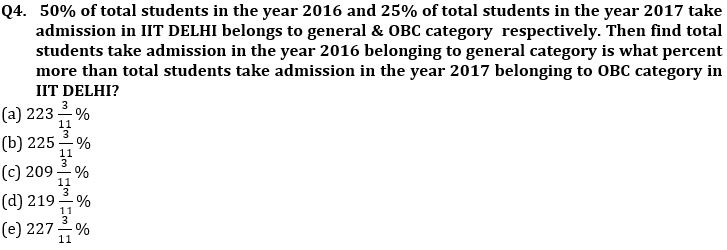Q5. 1/a+1/b=?
Quantity I: a & b are roots of equation x²+x-6=0
Quantity II: a²+b²=20;ab= 8
(a) Quantity I>Quantity II
(b) Quantity I≥Quantity II
(c) Quantity I<Quantity II
(d) Quantity I≤Quantity II
(e) Quantity I=Quantity II or no relation

Directions (6 – 9): Given radar chart shows the speed (in kmph) of 5 persons while going to any place and while returning to their initial position from that place. Read the data carefully and answer the questions.
(NOTE: All start from same position but they might go to same place or different place) (Speed of each person is uniform: (a) while going to any place and (b) while returning to their initial position from that place)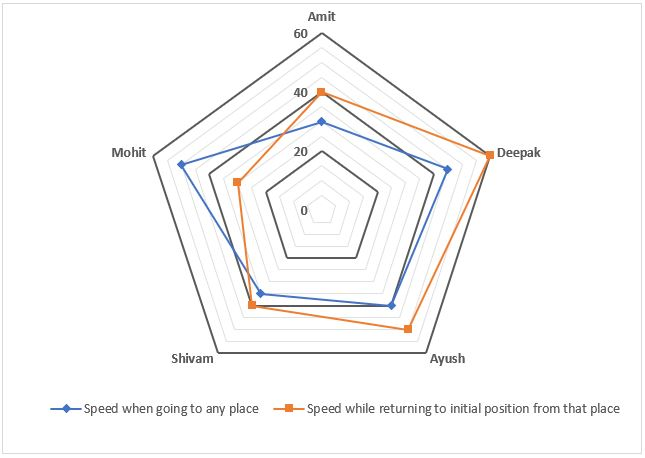Q6. If total time taken by Amit & Deepak for the entire round trip is same, then total distance travelled by Amit is what percent more/less than total distance travelled by Deepak during the entire round trip?
(a) 66⅔%
(b) 50%
(c) 25%
(d) 33⅓%
(e) 75%

Q7. What is the difference between average speed of Ayush & Mohit over their entire round trip? (approx.)
(a) 7 kmph
(b) 12 kmph
(c) None of these
(d) 5 kmph
(e) 9 kmph

Q8. If Ayush & Shivam both start at 7:00 AM to reach city X, which is 280 km away from the starting point and Ayush started returning to the starting point soon after reaching city X then at what approximate time will Ayush & Shivam meet?
(a) 2:32 PM
(b) 2:25 PM
(c) 3:12 PM
(d) 3:32 PM
(e) 2:36 PM

Q9. If Deepak & Mohit starts at same time to reach city Y, then what is the ratio of time taken by Deepak to that of by Mohit in reaching city Y?
(a) 48 : 35
(b) 35 : 48
(c) 9 : 10
(d) Cannot be determined
(e) 10 : 9

Q10. ‘X’ meters long train cross a pole in ‘t’ sec and a platform which is ‘L’ meters long in 20 sec. If speed of train is 72 km/hr, then what will be value of ‘t’ and ‘L’
(a) 16 & 140
(b) 12 & 200
(c) 8 & 240
(d) 8 & 200
(e) 16 & 120

Direction (11-15): Study the radar chart given below and answer the following questions.
Radar chart shows the markup % and discount % on five different articles sold by a shopkeeper.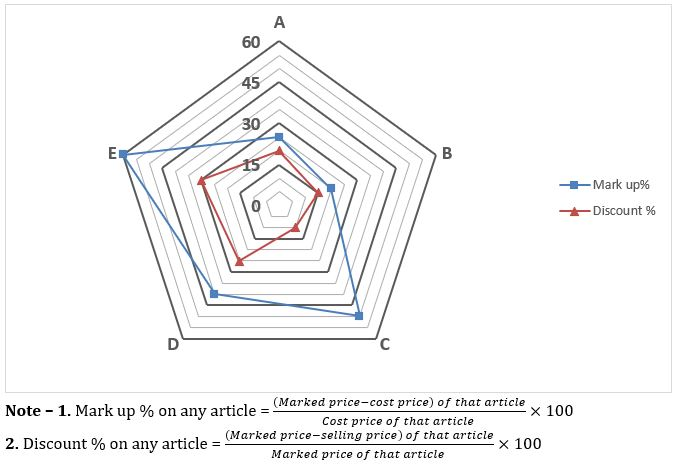Q11. If ratio of selling price of A to that of C is 40 : 27, then find marked price of C is what percent of cost price of A?
(a) 25%
(b) 100%
(c) 125%
(d) 50%
(e) 75%

Q12. If selling price of D is 40% more than marked price of B and selling price of B is Rs.290 less than cost price of D, then find the total profit earned by shopkeeper on selling 1 unit each of B & D?
(a) Rs.90
(b) Rs.50
(c) Rs.140
(d) Rs.150
(e) Rs.80

Q13. If shopkeeper earned total profit of Rs.36 on selling A & E, then find amount of discount allowed on E.
(a) Rs 144
(b) Rs 196
(c) Rs 158
(d) Cannot be determined
(e) None of the above.

Q14. If selling price of C & E together is Rs.2200 and ratio of cost price of C to selling price of E is 5 : 7, then find difference in marked prices of C & E.
(a) Rs.250
(b) Rs.450
(c) Rs.300
(d) Rs.400
(e) Rs.350

Q15. If selling price of A & B together is Rs.2330 and marked price of B is Rs.800 more than that of A, then find cost price of B is what percent of cost price of A?
(a) 137.5%
(b) 150%
(c) 162.5%
(d) 175%
(e) 187.5%

Solutions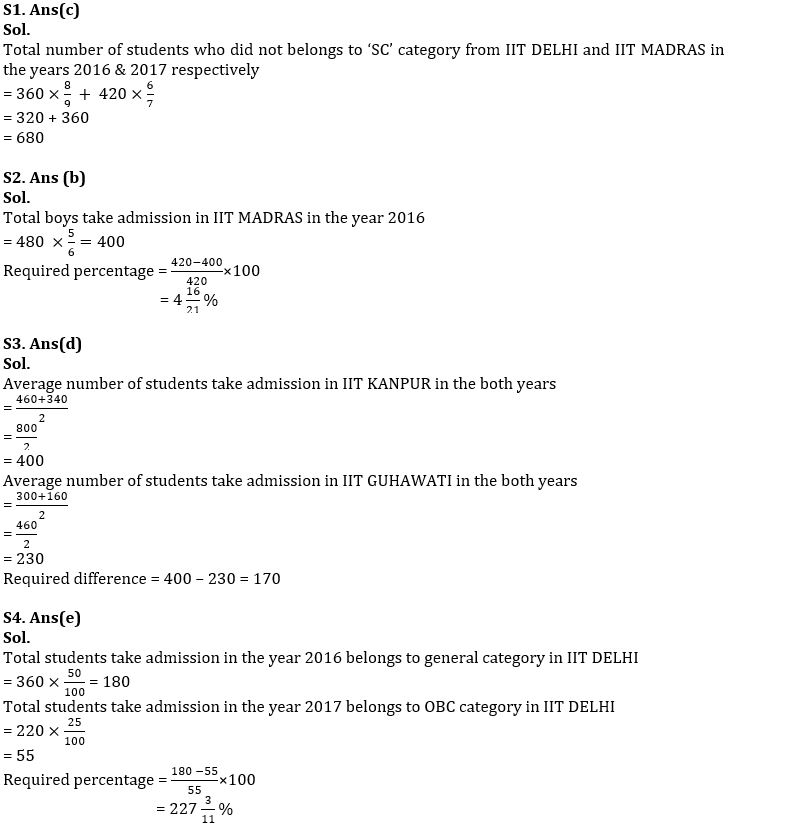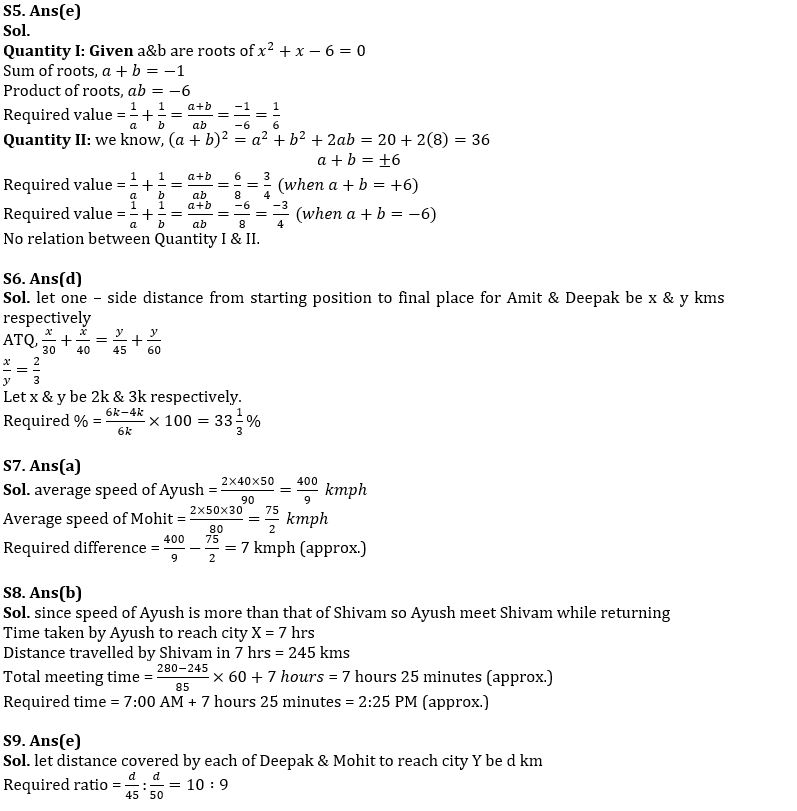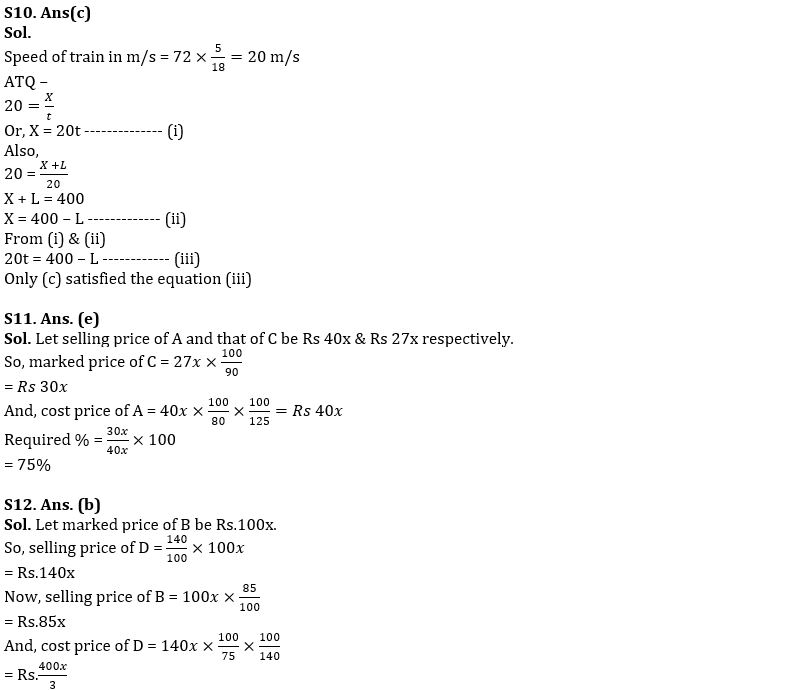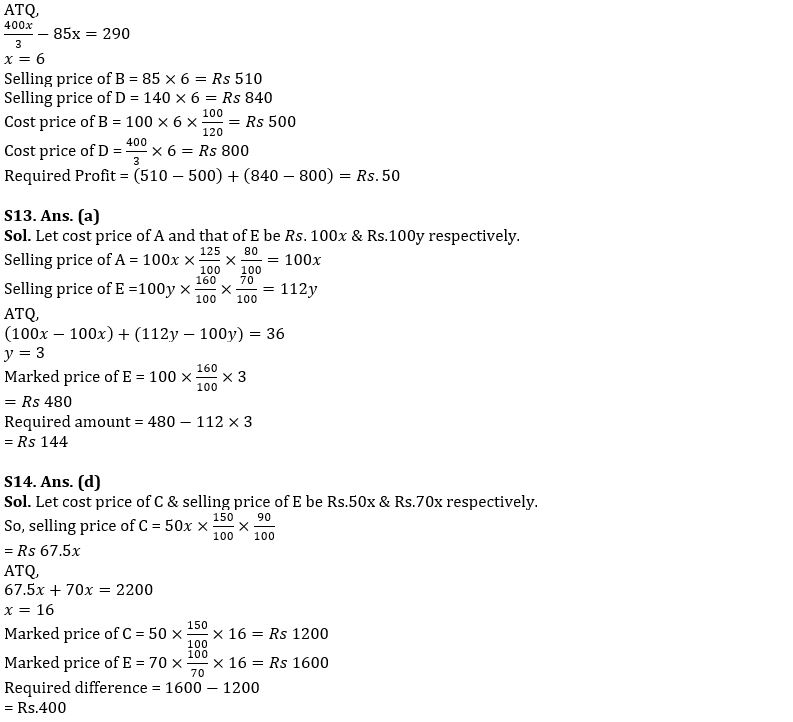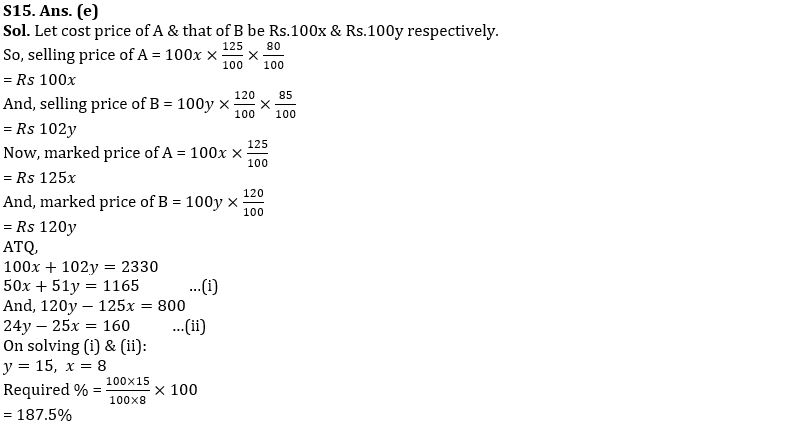#### Congratulations!Union Budget 2023-24: Free PDF